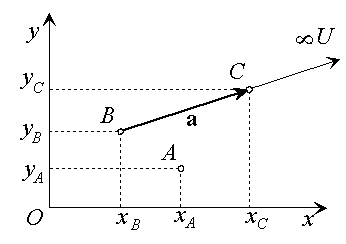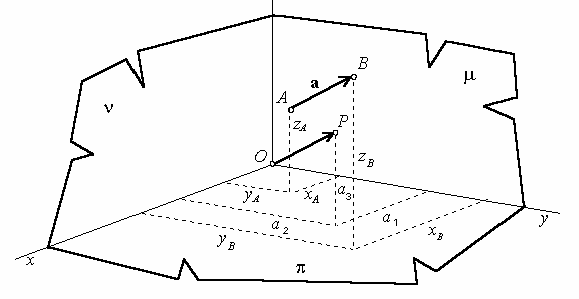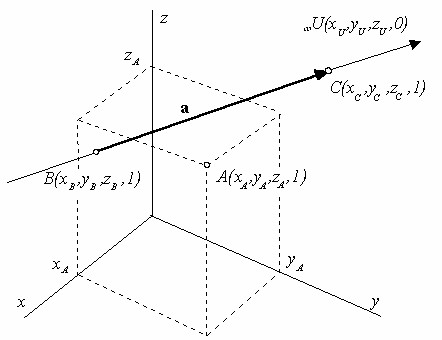# Homogeneous coordinates

Definition 1. Nonempty set ${\mathbf{P}}^{n}\left(R\right)$ is a projective space over the set of real numbers , iff

1. any point X in the space is attached a unique class $\left[x\right]$ of equivalent ordered sets of n+1 real numbers

 $\left({x}_{1},...,\phantom{\rule{mediummathspace}{0.2em}}{x}_{n},{x}_{0}\right)$

while two classes $\left({x}_{1},...,\phantom{\rule{mediummathspace}{0.2em}}{x}_{n},{x}_{0}\right)$ and $\left({y}_{1},...,\phantom{\rule{mediummathspace}{0.2em}}{y}_{n},{y}_{0}\right)$ are equivalent, if

 ${x}_{i}=h{y}_{i},\phantom{\rule{2mm}{2mm}}h\ne 0,\phantom{\rule{2mm}{2mm}}i=0,1,...,n$

2. class $\left(0,...,0\right)$ consisting of zeros only is attached to no point in the space.

Numbers ${x}_{0},\phantom{\rule{2mm}{2mm}}{x}_{1},\phantom{\rule{2mm}{2mm}}...,\phantom{\rule{2mm}{2mm}}{x}_{n}$ are homogeneous coordinates of the point in the projective space.

Arithmetic (algebraic) model of the n-dimensional projective space ${\mathbf{P}}^{n}\left(R\right)$ has the following properties:

1. There exist no more but at most (n +1) linearly independent points in the space.
2. All points in the space are linear combinations of any class of (n +1) linearly independent points. Space is therefore indicated also as linear space.
3. Set of points in the space that are linearly independent from (r +1) linearly independent points form the r-dimensional linear subspace ${\mathbf{P}}^{r}\left(R\right)$ of the space ${\mathbf{P}}^{n}\left(R\right)$.

Extended Euclidean plane ${{}_{\infty }\mathbf{E}}^{2}$ is model of the projective plane, and extended Euclidean space ${{}_{\infty }\mathbf{E}}^{3}\phantom{\rule{2mm}{2mm}}$is model of the projective space.

Homogeneous coordinate system in the projective space ${{}_{\infty }\mathbf{E}}^{2}$ is the extension of the Cartesian coordinate system in the Euclidean plane ${\mathbf{E}}^{2}\left(R\right)$.

Homogeneous coordinates of the point $A=\left({x}_{A},{y}_{A}\right)$ in the Euclidean subspace ${\mathbf{E}}^{2}\left(R\right)$ of the projective space ${{}_{\infty }\mathbf{E}}^{2}$ can be defined as any triple of real numbers $\left({a}_{1},{a}_{2},{a}_{0}\right),{a}_{0}\ne 0$, while the following holds

 ${x}_{A}=\frac{{a}_{1}}{{a}_{0}},\phantom{\rule{mediummathspace}{0.2em}}\phantom{\rule{mediummathspace}{0.2em}}{y}_{A}=\frac{{a}_{2}}{{a}_{0}}$

Any real point in the projective plane ${}_{\infty }{E}^{2}\phantom{\rule{2mm}{2mm}}$has the nonzero coordinate ${a}_{0}$.

Normal form of the homogeneous coordinates of the real point A is the triple of real numbers $A=\left({x}_{A},{y}_{A},1\right)$.Figure 1: Homogeneous coordinates of ideal point (direction)

Representative of a vector (direction) $\mathbf{a}=\stackrel{\to }{BC}$ is the oriented line segment determined by the real end points B and C. Homogeneous coordinates of the direction a representing the ideal point ${}_{\infty }U$ can be derived from the homogeneous coordinates of the chosen representative vector endpoints.

 $\mathbf{a}=\left({x}_{a},{y}_{a},0\right)=C-B=\left({x}_{C},{y}_{C},1\right)-\left({x}_{B},{y}_{B},1\right)=$
 $=\left({x}_{C}-{x}_{B},{y}_{C}-{y}_{B},0\right)=\left({x}_{U},{y}_{U},0\right)={}_{\infty }U$

Homogeneous coordinates of an ideal point in the projective plane ${}_{\infty }{E}^{2}\phantom{\rule{2mm}{2mm}}$form a triple of real numbers

 $\left({x}_{U},{y}_{U},0\right)=h\left({x}_{a},{y}_{a},0\right),h\ne 0$

where ${x}_{a},{y}_{a}$ are Cartesian coordinates of the ideal point (direction) chosen representative vector.

Homogeneous coordinates of the point $A=\left({x}_{A},{y}_{A},{z}_{A}\right)$ in the Euclidean subspace ${\mathbf{E}}^{3}\left(R\right)$ of the projective space ${{}_{\infty }\mathbf{E}}^{3}$ can be defined as any quadruple of real numbers $\left({a}_{1},{a}_{2},{a}_{3,}{a}_{0}\right),{a}_{0}\ne 0$, while the following holds

 ${x}_{A}=\frac{{a}_{1}}{{a}_{0}},\phantom{\rule{mediummathspace}{0.2em}}\phantom{\rule{mediummathspace}{0.2em}}{y}_{A}=\frac{{a}_{2}}{{a}_{0}},\phantom{\rule{2mm}{2mm}}\phantom{\rule{mediummathspace}{0.2em}}{z}_{A}=\frac{{a}_{3}}{{a}_{0}}$

Any real point in the projective space ${}_{\infty }{E}^{3}\phantom{\rule{2mm}{2mm}}$has the nonzero coordinate ${a}_{0}$.

Normal form of the homogeneous coordinates of the real point A is the quadruple of real numbers $A=\left({x}_{A},{y}_{A},{z}_{A},1\right)$.Figure 2: Coordinates of vector

Representative of a vector (direction) $\mathbf{a}=\stackrel{\to }{BC}$ is the oriented line segment determined by the real endpoints B and C. Homogeneous coordinates of the direction a representing the ideal point ${}_{\infty }U$ can be derived from the homogeneous coordinates of the chosen representative vector endpoints.

 $\mathbf{a}=\left({x}_{a},\phantom{\rule{2mm}{2mm}}{y}_{a},\phantom{\rule{2mm}{2mm}}{z}_{a},0\right)=C-B=\left({x}_{C},\phantom{\rule{2mm}{2mm}}{y}_{C},\phantom{\rule{2mm}{2mm}}{z}_{C},1\right)-\left({x}_{B},\phantom{\rule{2mm}{2mm}}{y}_{B},\phantom{\rule{2mm}{2mm}}{z}_{C},1\right)=$
 $=\left({x}_{C}-{x}_{B},{y}_{C}-{y}_{B},\phantom{\rule{2mm}{2mm}}{z}_{C}-{z}_{B},0\right)=\left({x}_{U},{y}_{U},\phantom{\rule{2mm}{2mm}}{z}_{U},0\right)={}_{\infty }U$

Homogeneous coordinates of the ideal point in the projective plane ${}_{\infty }{E}^{3}\phantom{\rule{2mm}{2mm}}$form a triple of real numbers

 $\left({x}_{U},{y}_{U},{z}_{U},0\right)=h\left({x}_{a},{y}_{a},{z}_{a},0\right),h\ne 0$

where ${x}_{a},{y}_{a},\phantom{\rule{2mm}{2mm}}{z}_{a}$ are Cartesian coordinates of the ideal point (direction) chosen representative.Figure 3: Homogeneous coordinates of ideal point